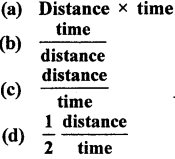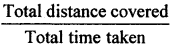## Physics ICSE Class 7 Important Questions Chapter 2 Motion

A. Tick (√) the following questions.

Question 1.
An object is said to be at rest if it does
(a) not move some time
(b) not move at all
(c) not breathe
(d) not move with respect to an observer
(d) not move with respect to an observer

Question 2.
Speed is –
(a) a physical quantity
(b) not a physical quantity
(c) a chemical quantity
(d) not a chemical quantity
(a) a physical quantity

Question 3.
Speed tells whether motion of an object is –
(a) slow
(b) fast
(c) (a)or(b)
(d) none of these
(c) (a) or (b)

Question 4.
Speedometer indicates speed of a vehicle which registered –
(a) every minute
(b) every hour
(c) at any instant
(d) every 5 minute
(c) at any instant

Question 5.
Speed is measured in –
(a) ms-1
(b) kmh-1
(c) ms
(d) any of (a) or (b)
(d) any of (a) or (b)

Question 6.
Formula of speed is –
(a) Distance x timeQuestion 7.
Mass of an object is the quantity of –
(a) matter contained in it
(b) matter outside it
(c) atoms in it
(d) molecules in it.
(a) matter contained in it

Question 8.
Mass of an object is a –
(a) constant quantity
(b) variable quantity
(c) any of (a) or (b)
(d) none of these
(a) constant quantity

Question 9.
Mass is measured in –
(a) m
(b) kg
(c) g
(d) any of (b) or (c)
(d) any of (b) or (c)

Question 10.
Mass is measured with –
(a) simple beam balance
(b) spring balance
(c) electronic balance
(d) (b)or(c)
(a) simple beam balance

Question 1.
Define Motion.
If an object changes its position with respect to its surroundings with time, it is said to be in motion.

Question 2.
Identify objects in motion and at rest.
Think that you are sitting in a standing bus. When you look out of the window you find that some trees in the rest state. But when the bus runs, the trees appear to be in motion. You are at rest when a person sitting with you in the bus sees you, but you are in motion along with the bus to a person standing on the road side. Thus it is clear that states of rest and motion are with respect to surroundings or a fixed point called reference point

Question 3.
Describe different types of motion, with examples from daily life.
Motion can be of following three types :
(a) Translatory motion : If all the points of an object change their place through the same distance in same time, then the object is said to be in translatory motion.
Examples :
1. An apple falling from a tree.
2. Ant moving in a straight line.

(b) Circular motion : If an object moves along a circle or circular path, then the object is said to be in circular motion.
Examples :
1. A running fan.
2. Hands of a clock. ,

(c) Oscillatory motion : If an object moves ‘to and fro’ from its position of rest, then the object is said to be in oscillatory motion.
Examples :
1. Motion of Pendulum of a wall clock.
2. Motion of a Swing.

Question 4.
Define uniform and non-uniform motion with examples from daily life.
Uniform motion : If an object covers equal distances in equal durations of time, then its motion is known as uniform motion.
Example : Hands of a clock or watch have uniform motion. Non-uniform
motion : If an object does not cover equal distances in equal intervals of time, then it is said to have nonuniform motion.
Example : Motion of a scooter.

Question 5.
Define concept of speed (average speed).
Average speed of an object is given by total distance travelled by the object divided by total time taken to cover the total
distance that is average Speed =Question 6.
Calculate average speed of objects based on data provided. Total distance covered
Average speed == $$\frac{(4+6+20) \mathrm{km}}{(5+5+15) \mathrm{min}}$$
= $$\frac{30 \mathrm{~km}}{25 \mathrm{~min}}$$
= $$\frac{30 \times 1000 \mathrm{~m}}{25 \times 60 \mathrm{~s}}$$
that is, average speed = 20 ms-1

Question 7.
Define weight.
Weight: It is the measure of the pull of Earth with which it attracts an object towards its centre.

Question 8.
Relate weight of an object with its mass.
Weight of an object = W
Mass of an object = M
Acceleration due to gravity = g W = Mg

C. Tick (√) the best choice :

Question 1.
A body can be –
(a) at rest relative to some object
(b) at motion relative to some object
(c) both (a) and (b)
(d) none of these
(c) both (a) and (b)

Question 2.
Every object in this universe is –
(a) in motion
(b) at rest
(c) any of (a) or (b)
(d) none of these
(c) any of (a) or (b)

Question 3.
If motion of a body is along a straight line, it is said to have –
(a) translatory motion
(b) rectilinear motion
(c) linear motion
(d) all of these
(b) rectilinear motion

Question 4.
Motion of pendulum is –
(a) linear
(b) vibratory
(c) oscillatory
(d) any of (b) or (c)
(d) any of (b) or (c)

Question 5.
Motion of hands of a clock is –
(a) uniform
(b) non-uniform
(c) any of (a) or (b)
(d) none of these
(a) uniform

D. Fill in the blanks :

Question 6.
If motion of an object is along a curve path, it is said to be …………… motion.
If motion of an object is along a curve path, it is said to be curvilinear motion.

Question 7.
Motion of Earth repeated after regular intervals of time …………… is called motion.
Motion of Earth repeated after regular intervals of time is called periodic motion.

Question 8.
When we breath our lungs repeatedly expand-contract, expand-contract. This motion is …………… motion.
When we breath our lungs repeatedly expand-contract, expand- contract. This motion is periodic motion.

Question 9.
Wheel of a running scooter has rotatory as well as ………….. motion.
Wheel of a running scooter has rotatory as well as circular motion.

Question 10.
Speed is given by distance travelled divided by ………………..
Speed is given by distance travelled divided by time taken.

E. Pick the true and false.

Question 11.
Mass of an object remains same everywhere.
True.

Question 12.
Weight of an object remains constant everywhere.
False.

Question 13.
Weight of an object is a force.
True.

Question 14.
Mass of an object is quantity of matter contained in it.
True.

Question 15.
Weight is measure in kg.
False.

F. Match the following :

Question 16.

 (a) Plucked string of guitar (i) linear (b) Beating of heartmn (ii) vibratory motion (c) Car moving on a straight road (iii) periodic motion (d) Car moving on a curved road (iv) curvilinear motion

 (a) Plucked string of guitar (ii) vibratory motion (b) Beating of heartmn (iii) periodic motion (c) Car moving on a straight road (i) linear (d) Car moving on a curved road (iv) curvilinear motion

G. Define :

Question 17.
Rotatory motion
If motion of an object is along its own axis then the motion of the object is called rotatory motion.

Question 18.
Oscillatory motion
If an object moves ‘to and fro’ from its position of rest, then the object is said to be in oscillatory motion.

Question 19.
Speed
Speed of an object is given by distance travelled by the object divided by time taken to cover the distance.
OR
Speed is defined as the distance covered by an object per unit time.

Question 20.
Weight
Weight is the measure of the pull of earth with which it attracts an object towards its centre.

H. Solve the following problems

Question 21.
A girl walks a distance of 2 km in 40 minute. Find her speed.
Distance = 2 km
1 km = 1000 m
2 km = 2 x 1000 = 2000 m
Time taken = 40 minutes
l minute = 60 seconds
40 minutes = 60 x 40 = 2400 seconds.Question 22.
if the girl in Q. 22. walk back and covers the same distance in 50 minute. What is the average speed of the girl.
Time taken = 50 minutes 1 minute = 60 seconds 50 minutes = 60 x 50 = 3000 seconds
Distance = 2000 m
Speed = $$\frac {2000}{3000}$$ = 0. 66 ms-1
Speed in Q. 21 = 0.83
Speed in Q. 22 = 0.66
Average speed = $$\frac {0.83 + 0.66}{2}$$
= $$\frac {1.49}{2}$$ = 0.74 ms-1

Question 23.
Weight of a body is 100 N. Find its mass.
Weight =100 N
g = 10 N/kg Mass = ?
Using, weight = Mass x g
We get, Mass = $$\frac {weight}{g}$$
= $$\frac {100 N}{10 N/kg}$$ = 10 kg

Question 24.
Mass of a body is 5 kg. Find its weight.